Publication date: 05/24/2021

## Power for Two Independent Sample Proportions

Use the Power Explorer for Two Independent Sample Proportions to determine a sample size for a hypothesis test for proportions from two groups. Select DOE > Sample Size Explorers > Power > Power for Two Independent Sample Proportions. Explore the trade offs between sample size, power, significance, and the hypothesized difference to detect. Sample size and power are associated with the following hypothesis test: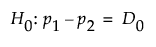versus the two-sided alternative: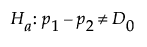or versus either of the following one-sided alternatives: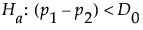or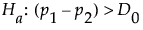where p1 and p2 are the population proportions from two populations, and D0 is the hypothesized difference in proportions.

### Power Explorer for Two Independent Sample Proportions Options

Set study assumptions and explore sample sizes using the radio buttons, text boxes, and sliders. The curve updates as you make changes to the settings. Alternatively, change settings by dragging the cross hairs on the curve or adjusting the values in the axis text boxes.

Test Type

Specifies a one or two-sided hypothesis test.

Fixed Parameters

Alpha

Specifies the probability of a type I error, which is the probability of rejecting the null hypothesis when it is true. It is commonly referred to as the significance level of the test. The default alpha level is 0.05.

Test Parameters

Parameters that are inter-related and update as you make changes.

Group 1proportion (p1)

Specifies the proportion that you anticipate or assume for Group 1.

Group 2 proportion (p2)

Specifies the proportion that you anticipate or assume for Group 2.

Group 1 Sample Size

Specifies the number of observations (runs, experimental units, or samples) needed for Group 1 in your experiment. Select Lock to lock the Group 1 sample size.

Group 2 Sample Size

Specifies the number of observations (runs, experimental units, or samples) needed for Group 2 in your experiment. Select Lock to lock the Group 2 sample size.

Total Sample Size

Specifies the total number of observations (runs, experimental units, or samples) needed for your experiment. The power curve is based on total sample size. Select Lock to lock the total sample size.

Note: Adjusting the sample size for one group adjusts the total sample size unless the total sample size is locked. In that case, adjusting the sample size for one group adjust the sample size for the second group. Adjusting the total sample size adjusts the two groups proportionally, unless one group is locked. Use only one lock at a time.

Power

Specifies the probability of rejecting the null hypothesis when it is false. With all other parameters fixed, power increases as sample size increases.

Save Settings

Saves the current settings to the Saved Settings table. This enables you to save a set of alternative study plans. See Saved Settings in the Sample Size Explorers.

Make Data Collection Table

Creates a new data table that you can use for data collection.

### Statistical Details for Two Independent Sample Proportions

The power calculations for testing the difference in proportions from two sample groups are based on the normal approximation. The calculations depend on the form of the alternative hypothesis. For a one-sided, higher alternative: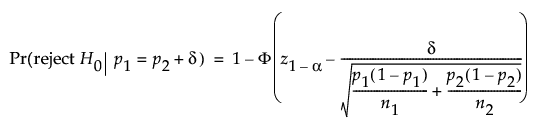For a one-sided, lower alternative: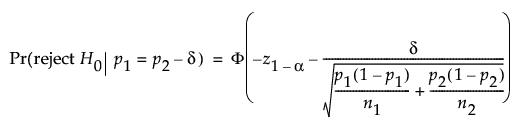For a two-sided alternative: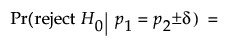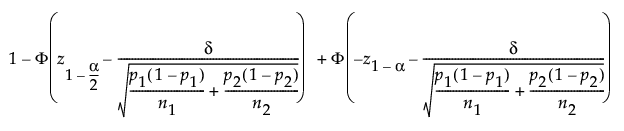where:

α is the significance level

n1 and n2 are the group sample sizes

p1 and p2 are the group proportions

δ is the difference to detect

z1-α is the (1 - α)th quantile of the z-distribution

Φ(x) is the cumulative distribution function of the normal distribution.